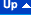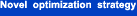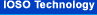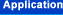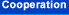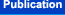Effective Approach for Multidisciplinary Optimization Problems Solution

UEF, Optimization in Industry III, Italy, 2001.

Nowadays efficient procedures development for real-life multidisciplinary optimization problems solution is a problem of today. The reduction of multidisciplinary optimization problem solution time can be achieved by means of:

i) an approximation technology usage;

ii) an optimization process parallelizing;

iii) a multilevel approach usage for researches;

In the present paper there is being discussed a new approach for multidisciplinary optimization problems solution. It is based upon the adaptive usage of the three above-noted technologies (i, ii, iii). This fact allows us to insure the high efficiency of computing process.

i) The Usage of Approximation technology.

The approximation technology is being implemented as the method of indirect optimization which is based on self-organization (IOSO). The main peculiarities of our multiparameter approximation technology are the following.

The IOSO is based upon the use of self-organization and evolutionary modeling principles to correct iteratively the structure and the parameters of the response surface approximation in the process of extremum search. The distinctive feature of this approach consists in the fact that it requires extremely low number of trial points in the experiment plan to initialize the algorithm (30...50 data points for the optimization problems with nearly 100 variables dimensionality).

The main feature of this approach is the decomposition of the approximation function into the set of the simple approximation functions. The final response function is the multilevel graph. The total power of final polynomial can be high enough and is determined in the process of evolutionary synthesis of the response function structure.

The obtained response surface functions are naturally used in the optimization procedures. While passing from one iteration to another, the following steps are carried out:

• modification of experiment plan;
• adaptive selection of current extremum search area;
• choice of the response function type (global or middle-range);
• transformation of the response function;
• modification of both parameters and structure of the optimization algorithms;
• if necessary, selection of the new promising points within the search area.

ii) The optimization process parallelizing.

The effectiveness of parallel computations usage for optimization process based on multipoint approximation is obvious . Generally, by approximation technology use, this procedure consists in the parallel computation of the object function values at the stage of experiment plan formation. As a whole it insures the reduction of CPU time for solution of optimization problems.

The usage of computations parallelizing technology in the very optimization procedure is more efficient. It means that this technology usage leads to the changes of:

a) the computational process within the frameworks of a single iteration;

b) the moving strategy.

Main peculiarities of our new procedure include the building up approximation functions set at every iteration of optimization process. These functions differ according to the areas of the best approximation, complexity and accuracy. Then, from the whole set of the generated approximation functions there is being chosen some set of the best functions according to the accuracy and stability criteria. The regularization procedure is used at this stage. In accordance with different properties of this set of the best approximation functions there is being performed the change of moving strategy parameters and structure. It is insured by means of the adaptive evolutionary procedure usage. Each of the obtained best approximation functions is being optimized.

Thus, at each iteration of the optimization process there is being determined the set of perspective points. The object function is being computed for these points only. The parallel computations technology is also used to determine the set of the object function true values.

The set of these new points is used for the experiment plan modification, the search region adaptation and change of optimization algorithm parameters and structure.

In the paper there are cited the numerical researches results with different CPUs numbers and the optimization problems dimensionalities (up to 80). It is demonstrated that the usage of multiparallel optimization procedure can allow us to reduce CPU time more greatly than the number of CPUs. It proves that the given optimization procedure has been developed according to the parallel optimization process, and that is not a trivial usage of several CPUs for optimization problems solution.

iii) The usage of multilevel mathematical model for researches.

Multilevel procedures allow us to use additional resources to reduce CPU time for optimization researches. It is possible to use different versions of multilevel procedures:

1) multilevel analysis tool use;

2) multilevel approximation model use;

3) the combination of i) and ii).

The present paper considers the procedure of multicriteria optimization of complicated systems based upon the adaptive use of various levels of analysis tools. The offered approach is intended to minimize the use of complicated tools of the analysis. It ensures the search possibilities for Pareto-optimal set of solutions, and it also ensures improvements of the lower level mathematical model.

The simplified scheme of such a procedure operation can be represented as follows. The multicriteria optimization problem is solved basing upon the simplified mathematical model. As a result we obtain the set of Pareto-optimal solutions which are uniformly distributed in the design space according to optimization criteria. Then for the obtained Pareto set the indicators of effectiveness are updated by using the high-fidelity analysis tools. The identification of the simplified mathematical model is being performed. The simplified mathematical model is being replaced by the identified one and the procedure is being repeated.

The information stored during the search is used to improve the simplified models. After the termination of the given procedure one can construct the response functions of the researched object. However, both identification and approximation are not fitted for the whole initial search area, but only for some neighborhood of the obtained Pareto set. It ensures purposeful improvement of approximating properties just in the area of optimal solutions, that noticeably reduces the laboriousness to construct these functions.

The developed approaches considerably increase the effectiveness of complicated multicriteria optimization problems solution. The stored information for identification of the simplified tools and for approximation allows us to expand hierarchically the thoroughness of the problem review. It also allows us to add some new disciplines for the analysis.

In the present paper there are presented the research results for test problems as well as for the real-life optimization problems with 20...80 design variables (for optimal design of aircraft engine).